# kinetics (Lec 2).pptx

19 de Mar de 2023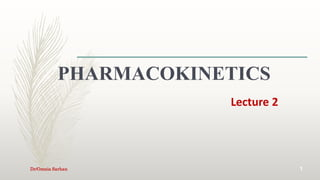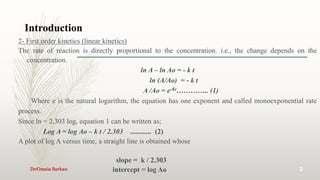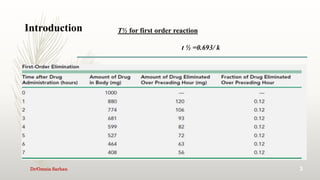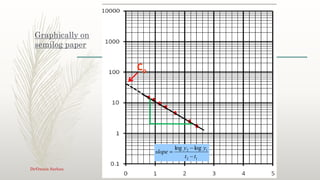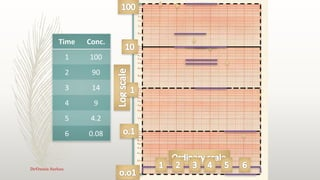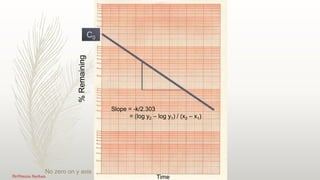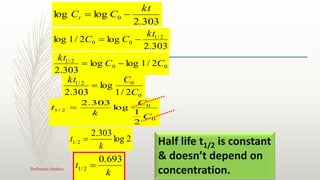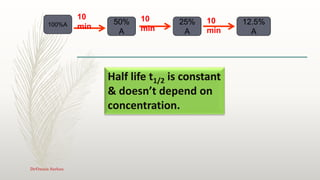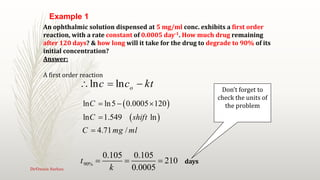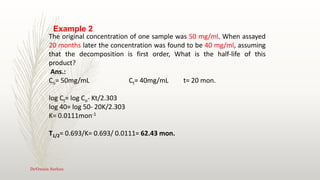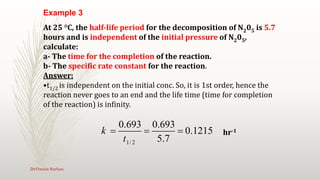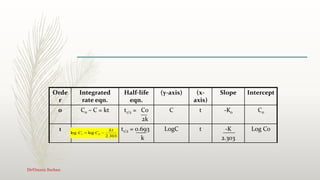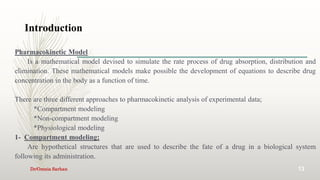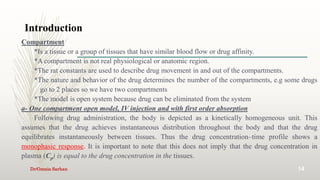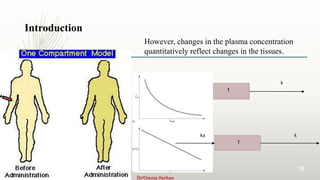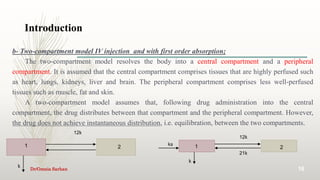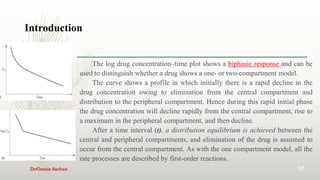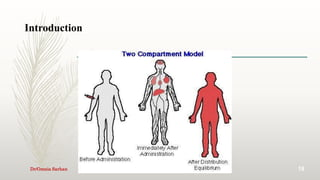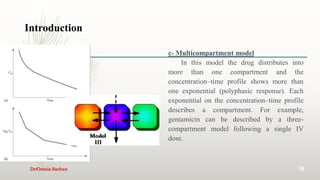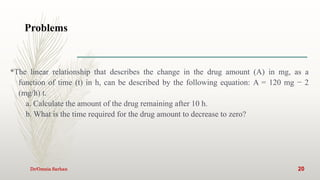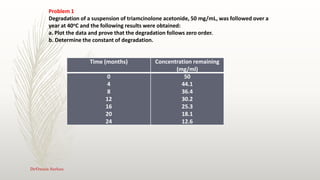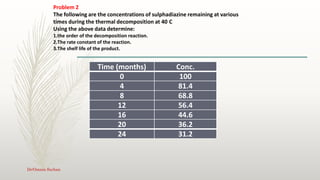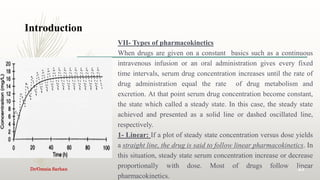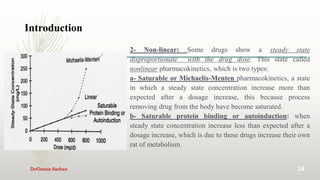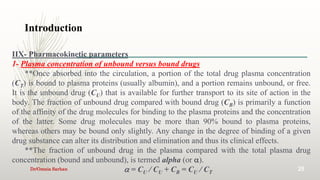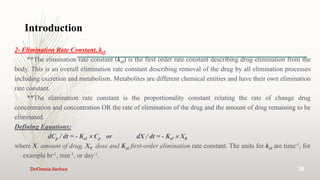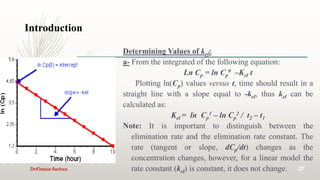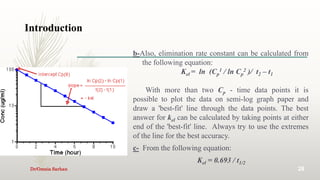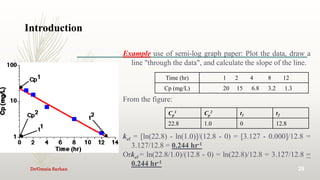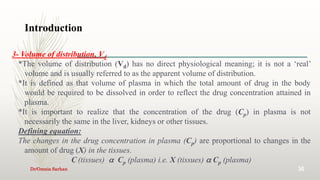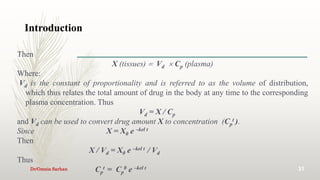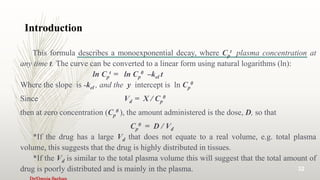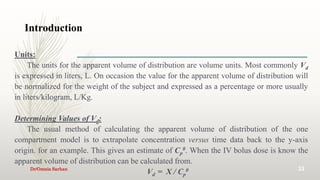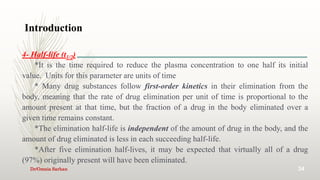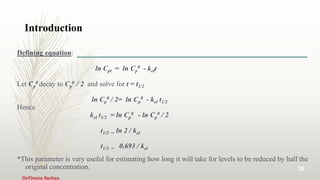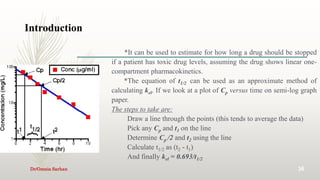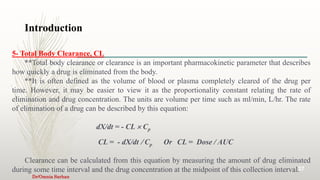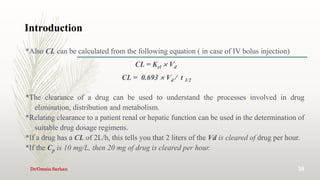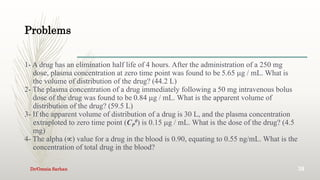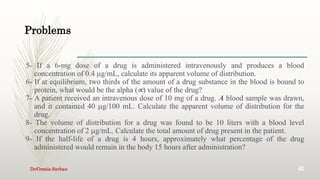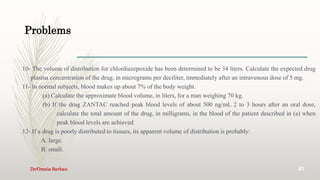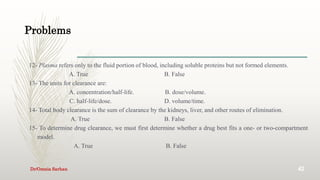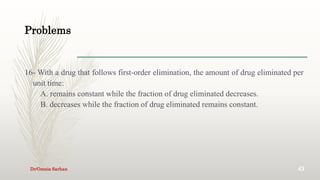1 de 44

### kinetics (Lec 2).pptx

• 2. Introduction 2 2- First order kinetics (linear kinetics) The rate of reaction is directly proportional to the concentration. i.e., the change depends on the concentration. ln A – ln Ao = - k t ln (A/Ao) = - k t A /Ao = e-Kt………….. (1) Where e is the natural logarithm, the equation has one exponent and called monoexponential rate process. Since ln = 2.303 log, equation 1 can be written as; Log A = log Ao – k t / 2.303 ............ (2) A plot of log A versus time, a straight line is obtained whose slope = k / 2.303 intercept = log Ao Dr/Omnia Sarhan
• 3. Introduction 3 T½ for first order reaction t ½ =0.693/ k Dr/Omnia Sarhan
• 4. Graphically on semilog paper Co 1 2 1 2 log log t t y y slope    4 Dr/Omnia Sarhan
• 6. % Remaining Time C0 Slope = -k/2.303 = (log y2 – log y1) / (x2 – x1) No zero on y axis 6 Dr/Omnia Sarhan
• 7. k t 693 . 0 2 / 1  Half life t1/2 is constant & doesn’t depend on concentration. 2 log 303 . 2 2 / 1 k t  303 . 2 log log 0 kt C Ct   303 . 2 log 2 / 1 log 2 / 1 0 0 kt C C   0 0 2 / 1 2 1 log 303 . 2 C C k t  0 0 2 / 1 2 / 1 log log 303 . 2 C C kt   0 0 2 / 1 2 / 1 log 303 . 2 C C kt  7 Dr/Omnia Sarhan
• 8. 100%A 10 min 50% A 25% A 12.5% A 10 min 10 min Half life t1/2 is constant & doesn’t depend on concentration. 8 Dr/Omnia Sarhan
• 9. ln ln o c c kt        ln ln5 0.0005 120 ln 1.549 ln 4.71 / C C shift C mg ml      90% 0.105 0.105 210 0.0005 t k    Don’t forget to check the units of the problem An ophthalmic solution dispensed at 5 mg/ml conc. exhibits a first order reaction, with a rate constant of 0.0005 day-1. How much drug remaining after 120 days? & how long will it take for the drug to degrade to 90% of its initial concentration? Answer: A first order reaction days Example 1 9 Dr/Omnia Sarhan
• 10. The original concentration of one sample was 50 mg/ml. When assayed 20 months later the concentration was found to be 40 mg/ml, assuming that the decomposition is first order, What is the half-life of this product? Ans.: Co= 50mg/mL Ct= 40mg/mL t= 20 mon. log Ct= log Co- Kt/2.303 log 40= log 50- 20K/2.303 K= 0.0111mon-1 T1/2= 0.693/K= 0.693/ 0.0111= 62.43 mon. Example 2 10 Dr/Omnia Sarhan
• 11. 1/ 2 0.693 0.693 0.1215 5.7 k t    At 25 °C, the half-life period for the decomposition of N205 is 5.7 hours and is independent of the initial pressure of N205, calculate: a- The time for the completion of the reaction. b- The specific rate constant for the reaction. Answer: •t1/2 is independent on the initial conc. So, it is 1st order, hence the reaction never goes to an end and the life time (time for completion of the reaction) is infinity. hr-1 Example 3 11 Dr/Omnia Sarhan
• 12. Intercept Slope (x- axis) (y-axis) Half-life eqn. Integrated rate eqn. Orde r Co -Ko t C t1/2 = Co 2k Co – C = kt 0 Log Co -K 2.303 t LogC t1/2 = 0.693 k 1 303 . 2 log log 0 kt C Ct   Dr/Omnia Sarhan
• 13. Introduction 13 Pharmacokinetic Model Is a mathematical model devised to simulate the rate process of drug absorption, distribution and elimination. These mathematical models make possible the development of equations to describe drug concentration in the body as a function of time. There are three different approaches to pharmacokinetic analysis of experimental data; *Compartment modeling *Non-compartment modeling *Physiological modeling 1- Compartment modeling; Are hypothetical structures that are used to describe the fate of a drug in a biological system following its administration. Dr/Omnia Sarhan
• 14. Introduction 14 Compartment *Is a tissue or a group of tissues that have similar blood flow or drug affinity. *A compartment is not real physiological or anatomic region. *The rat constants are used to describe drug movement in and out of the compartments. *The nature and behavior of the drug determines the number of the compartments, e.g some drugs go to 2 places so we have two compartments *The model is open system because drug can be eliminated from the system a- One compartment open model, IV injection and with first order absorption Following drug administration, the body is depicted as a kinetically homogeneous unit. This assumes that the drug achieves instantaneous distribution throughout the body and that the drug equilibrates instantaneously between tissues. Thus the drug concentration–time profile shows a monophasic response. It is important to note that this does not imply that the drug concentration in plasma (Cp) is equal to the drug concentration in the tissues. Dr/Omnia Sarhan
• 15. Introduction 15 k 1 However, changes in the plasma concentration quantitatively reflect changes in the tissues. k 1 ka Dr/Omnia Sarhan
• 16. Introduction 16 b- Two-compartment model IV injection and with first order absorption; The two-compartment model resolves the body into a central compartment and a peripheral compartment. It is assumed that the central compartment comprises tissues that are highly perfused such as heart, lungs, kidneys, liver and brain. The peripheral compartment comprises less well-perfused tissues such as muscle, fat and skin. A two-compartment model assumes that, following drug administration into the central compartment, the drug distributes between that compartment and the peripheral compartment. However, the drug does not achieve instantaneous distribution, i.e. equilibration, between the two compartments. k 12 1 2 k k 12 1 2 k k 21 ka Dr/Omnia Sarhan
• 17. Introduction 17 The log drug concentration–time plot shows a biphasic response and can be used to distinguish whether a drug shows a one- or two-compartment model. The curve shows a profile in which initially there is a rapid decline in the drug concentration owing to elimination from the central compartment and distribution to the peripheral compartment. Hence during this rapid initial phase the drug concentration will decline rapidly from the central compartment, rise to a maximum in the peripheral compartment, and then decline. After a time interval (t), a distribution equilibrium is achieved between the central and peripheral compartments, and elimination of the drug is assumed to occur from the central compartment. As with the one compartment model, all the rate processes are described by first-order reactions. Dr/Omnia Sarhan
• 19. Introduction 19 c- Multicompartment model In this model the drug distributes into more than one compartment and the concentration–time profile shows more than one exponential (polyphasic response). Each exponential on the concentration–time profile describes a compartment. For example, gentamicin can be described by a three- compartment model following a single IV dose. Dr/Omnia Sarhan
• 20. Problems Dr/Omnia Sarhan 20 *The linear relationship that describes the change in the drug amount (A) in mg, as a function of time (t) in h, can be described by the following equation: A = 120 mg − 2 (mg/h) t. a. Calculate the amount of the drug remaining after 10 h. b. What is the time required for the drug amount to decrease to zero?
• 21. Time (months) Concentration remaining (mg/ml) 0 4 8 12 16 20 24 50 44.1 36.4 30.2 25.3 18.1 12.6 Problem 1 Degradation of a suspension of triamcinolone acetonide, 50 mg/mL, was followed over a year at 40oC and the following results were obtained: a. Plot the data and prove that the degradation follows zero order. b. Determine the constant of degradation. 21 Dr/Omnia Sarhan
• 22. Time (months) Conc. 0 100 4 81.4 8 68.8 12 56.4 16 44.6 20 36.2 24 31.2 Problem 2 The following are the concentrations of sulphadiazine remaining at various times during the thermal decomposition at 40 C Using the above data determine: 1.the order of the decomposition reaction. 2.The rate constant of the reaction. 3.The shelf life of the product. Dr/Omnia Sarhan
• 23. Introduction 23 VII- Types of pharmacokinetics When drugs are given on a constant basics such as a continuous intravenous infusion or an oral administration gives every fixed time intervals, serum drug concentration increases until the rate of drug administration equal the rate of drug metabolism and excretion. At that point serum drug concentration become constant, the state which called a steady state. In this case, the steady state achieved and presented as a solid line or dashed oscillated line, respectively. 1- Linear: If a plot of steady state concentration versus dose yields a straight line, the drug is said to follow linear pharmacokinetics. In this situation, steady state serum concentration increase or decrease proportionally with dose. Most of drugs follow linear pharmacokinetics. Dr/Omnia Sarhan
• 24. Introduction 24 2- Non-linear: Some drugs show a steady state disproportionate with the drug dose. This state called nonlinear pharmacokinetics, which is two types: a- Saturable or Michaelis-Menten pharmacokinetics, a state in which a steady state concentration increase more than expected after a dosage increase, this because process removing drug from the body have become saturated. b- Saturable protein binding or autoinduction: when steady state concentration increase less than expected after a dosage increase, which is due to these drugs increase their own rat of metabolism. Dr/Omnia Sarhan
• 25. Introduction 25 IIX- Pharmacokinetic parameters 1- Plasma concentration of unbound versus bound drugs **Once absorbed into the circulation, a portion of the total drug plasma concentration (CT) is bound to plasma proteins (usually albumin), and a portion remains unbound, or free. It is the unbound drug (CU) that is available for further transport to its site of action in the body. The fraction of unbound drug compared with bound drug (CB) is primarily a function of the affinity of the drug molecules for binding to the plasma proteins and the concentration of the latter. Some drug molecules may be more than 90% bound to plasma proteins, whereas others may be bound only slightly. Any change in the degree of binding of a given drug substance can alter its distribution and elimination and thus its clinical effects. **The fraction of unbound drug in the plasma compared with the total plasma drug concentration (bound and unbound), is termed alpha (or ).  = CU / CU + CB = CU / CT Dr/Omnia Sarhan
• 26. Introduction 26 2- Elimination Rate Constant, kel **The elimination rate constant (kel) is the first order rate constant describing drug elimination from the body. This is an overall elimination rate constant describing removal of the drug by all elimination processes including excretion and metabolism. Metabolites are different chemical entities and have their own elimination rate constant. **The elimination rate constant is the proportionality constant relating the rate of change drug concentration and concentration OR the rate of elimination of the drug and the amount of drug remaining to be eliminated. Defining Equations: dCp / dt = - Kel  Cp or dX / dt = - Kel  X0 where X amount of drug, X0 dose and Kel first-order elimination rate constant. The units for kel are time-1, for example hr-1, min-1, or day-1. Dr/Omnia Sarhan
• 27. Introduction 27 Determining Values of kel: a- From the integrated of the following equation: Ln Cp = ln Cp 0 –Kel t Plotting ln(Cp) values versus t, time should result in a straight line with a slope equal to -kel, thus kel can be calculated as: Kel = ln Cp 1 – ln Cp 2 / t2 – t1 Note: It is important to distinguish between the elimination rate and the elimination rate constant. The rate (tangent or slope, dCp/dt) changes as the concentration changes, however, for a linear model the rate constant (kel) is constant, it does not change. Dr/Omnia Sarhan
• 28. Introduction 28 b-Also, elimination rate constant can be calculated from the following equation: Kel = ln (Cp 1 / ln Cp 2 )/ t2 – t1 With more than two Cp - time data points it is possible to plot the data on semi-log graph paper and draw a 'best-fit' line through the data points. The best answer for kel can be calculated by taking points at either end of the 'best-fit' line. Always try to use the extremes of the line for the best accuracy. c- From the following equation: Kel = 0.693 / t1/2 Dr/Omnia Sarhan
• 29. Introduction 29 Example use of semi-log graph paper: Plot the data, draw a line "through the data", and calculate the slope of the line. From the figure: kel = [ln(22.8) - ln(1.0)]/(12.8 - 0) = [3.127 - 0.000]/12.8 = 3.127/12.8 = 0.244 hr-1 Orkel = ln(22.8/1.0)/(12.8 - 0) = ln(22.8)/12.8 = 3.127/12.8 = 0.244 hr-1 1 2 4 8 12 Time (hr) 20 15 6.8 3.2 1.3 Cp (mg/L) t2 t1 Cp 2 Cp 1 12.8 0 1.0 22.8 Dr/Omnia Sarhan
• 30. Introduction 30 3- Volume of distribution, Vd *The volume of distribution (Vd) has no direct physiological meaning; it is not a ‘real’ volume and is usually referred to as the apparent volume of distribution. *It is defined as that volume of plasma in which the total amount of drug in the body would be required to be dissolved in order to reflect the drug concentration attained in plasma. *It is important to realize that the concentration of the drug (Cp) in plasma is not necessarily the same in the liver, kidneys or other tissues. Defining equation: The changes in the drug concentration in plasma (Cp) are proportional to changes in the amount of drug (X) in the tissues. C (tissues)  Cp (plasma) i.e. X (tissues)  Cp (plasma) Dr/Omnia Sarhan
• 31. Introduction 31 Then X (tissues)  Vd  Cp (plasma) Where: Vd is the constant of proportionality and is referred to as the volume of distribution, which thus relates the total amount of drug in the body at any time to the corresponding plasma concentration. Thus Vd = X / Cp and Vd can be used to convert drug amount X to concentration (Cp t ). Since X = X0 e –kel t Then X / Vd = X0 e –kel t / Vd Thus Cp t = Cp 0 e –kel t Dr/Omnia Sarhan
• 32. Introduction 32 This formula describes a monoexponential decay, where Cp t plasma concentration at any time t. The curve can be converted to a linear form using natural logarithms (ln): ln Cp t = ln Cp 0 –kel t Where the slope is -kel , and the y intercept is ln Cp 0 Since Vd = X / Cp 0 then at zero concentration (Cp 0 ), the amount administered is the dose, D, so that Cp 0 = D / Vd *If the drug has a large Vd that does not equate to a real volume, e.g. total plasma volume, this suggests that the drug is highly distributed in tissues. *If the Vd is similar to the total plasma volume this will suggest that the total amount of drug is poorly distributed and is mainly in the plasma.
• 33. Introduction 33 Units: The units for the apparent volume of distribution are volume units. Most commonly Vd is expressed in liters, L. On occasion the value for the apparent volume of distribution will be normalized for the weight of the subject and expressed as a percentage or more usually in liters/kilogram, L/Kg. Determining Values of Vd: The usual method of calculating the apparent volume of distribution of the one compartment model is to extrapolate concentration versus time data back to the y-axis origin. for an example. This gives an estimate of Cp 0. When the IV bolus dose is know the apparent volume of distribution can be calculated from. Vd = X / Cp 0 Dr/Omnia Sarhan
• 34. Introduction 34 4- Half-life (t1/2) *It is the time required to reduce the plasma concentration to one half its initial value. Units for this parameter are units of time * Many drug substances follow first-order kinetics in their elimination from the body, meaning that the rate of drug elimination per unit of time is proportional to the amount present at that time, but the fraction of a drug in the body eliminated over a given time remains constant. *The elimination half-life is independent of the amount of drug in the body, and the amount of drug eliminated is less in each succeeding half-life. *After five elimination half-lives, it may be expected that virtually all of a drug (97%) originally present will have been eliminated. Dr/Omnia Sarhan
• 35. Introduction 35 Defining equation: ln Cpt = ln Cp 0 - kelt Let Cp 0 decay to Cp 0 / 2 and solve for t = t1/2 ln Cp 0 / 2= ln Cp 0 - kel t1/2 Hence kel t1/2 = ln Cp 0 - ln Cp 0 / 2 t1/2 = ln 2 / kel t1/2 = 0.693 / kel *This parameter is very useful for estimating how long it will take for levels to be reduced by half the original concentration. Dr/Omnia Sarhan
• 36. Introduction 36 *It can be used to estimate for how long a drug should be stopped if a patient has toxic drug levels, assuming the drug shows linear one- compartment pharmacokinetics. *The equation of t1/2 can be used as an approximate method of calculating kel. If we look at a plot of Cp versus time on semi-log graph paper. The steps to take are: Draw a line through the points (this tends to average the data) Pick any Cp and t1 on the line Determine Cp /2 and t2 using the line Calculate t1/2 as (t2 - t1) And finally kel = 0.693/t1/2 Dr/Omnia Sarhan
• 37. Introduction 37 5- Total Body Clearance, CL **Total body clearance or clearance is an important pharmacokinetic parameter that describes how quickly a drug is eliminated from the body. **It is often defined as the volume of blood or plasma completely cleared of the drug per time. However, it may be easier to view it as the proportionality constant relating the rate of elimination and drug concentration. The units are volume per time such as ml/min, L/hr. The rate of elimination of a drug can be described by this equation: dX/dt = - CL  Cp CL = - dX/dt  Cp Or CL = Dose / AUC Clearance can be calculated from this equation by measuring the amount of drug eliminated during some time interval and the drug concentration at the midpoint of this collection interval. Dr/Omnia Sarhan
• 38. Introduction 38 *Also CL can be calculated from the following equation ( in case of IV bolus injection) CL = Kel  Vd CL = 0.693  Vd / t 1/2 *The clearance of a drug can be used to understand the processes involved in drug elimination, distribution and metabolism. *Relating clearance to a patient renal or hepatic function can be used in the determination of suitable drug dosage regimens. *If a drug has a CL of 2L/h, this tells you that 2 liters of the Vd is cleared of drug per hour. *If the Cp is 10 mg/L, then 20 mg of drug is cleared per hour. Dr/Omnia Sarhan
• 39. Problems 39 1- A drug has an elimination half life of 4 hours. After the administration of a 250 mg dose, plasma concentration at zero time point was found to be 5.65 g / mL. What is the volume of distribution of the drug? (44.2 L) 2- The plasma concentration of a drug immediately following a 50 mg intravenous bolus dose of the drug was found to be 0.84 g / mL. What is the apparent volume of distribution of the drug? (59.5 L) 3- If the apparent volume of distribution of a drug is 30 L, and the plasma concentration extraploted to zero time point (CP 0) is 0.15 g / mL. What is the dose of the drug? (4.5 mg) 4- The alpha () value for a drug in the blood is 0.90, equating to 0.55 ng/mL. What is the concentration of total drug in the blood? Dr/Omnia Sarhan
• 40. Problems 40 5- If a 6-mg dose of a drug is administered intravenously and produces a blood concentration of 0.4 g/mL, calculate its apparent volume of distribution. 6- If at equilibrium, two thirds of the amount of a drug substance in the blood is bound to protein, what would be the alpha () value of the drug? 7- A patient received an intravenous dose of 10 mg of a drug. A blood sample was drawn, and it contained 40 g/100 mL. Calculate the apparent volume of distribution for the drug. 8- The volume of distribution for a drug was found to be 10 liters with a blood level concentration of 2 g/mL. Calculate the total amount of drug present in the patient. 9- If the half-life of a drug is 4 hours, approximately what percentage of the drug administered would remain in the body 15 hours after administration? Dr/Omnia Sarhan
• 41. Problems 41 10- The volume of distribution for chlordiazepoxide has been determined to be 34 liters. Calculate the expected drug plasma concentration of the drug, in micrograms per deciliter, immediately after an intravenous dose of 5 mg. 11- In normal subjects, blood makes up about 7% of the body weight. (a) Calculate the approximate blood volume, in liters, for a man weighing 70 kg. (b) If the drug ZANTAC reached peak blood levels of about 500 ng/mL 2 to 3 hours after an oral dose, calculate the total amount of the drug, in milligrams, in the blood of the patient described in (a) when peak blood levels are achieved 12- If a drug is poorly distributed to tissues, its apparent volume of distribution is probably: A. large. B. small. Dr/Omnia Sarhan
• 42. Problems 42 12- Plasma refers only to the fluid portion of blood, including soluble proteins but not formed elements. A. True B. False 13- The units for clearance are: A. concentration/half-life. B. dose/volume. C. half-life/dose. D. volume/time. 14- Total body clearance is the sum of clearance by the kidneys, liver, and other routes of elimination. A. True B. False 15- To determine drug clearance, we must first determine whether a drug best fits a one- or two-compartment model. A. True B. False Dr/Omnia Sarhan
• 43. Problems 43 16- With a drug that follows first-order elimination, the amount of drug eliminated per unit time: A. remains constant while the fraction of drug eliminated decreases. B. decreases while the fraction of drug eliminated remains constant. Dr/Omnia Sarhan